International
Tables for
Crystallography
Volume B
Reciprocal space
Edited by U. Shmueli

International Tables for Crystallography (2006). Vol. B. ch. 2.5, pp. 309-310   | 1 | 2 |

## Section 2.5.4.4. Structure analysis

B. K. Vainshteinc and B. B. Zvyagind

#### 2.5.4.4. Structure analysis

| top | pdf |

The unit cell is defined on the basis of the geometric theory of electron-diffraction patterns, and the space group from extinctions. It is also possible to use the method of converging beams (Section 5.2.2). The structural determination is based on experimental sets of values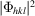or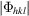(Vainshtein, 1964).

The trial-and-error method may be used for the simplest structures. The main method of determination is the construction of the Patterson functions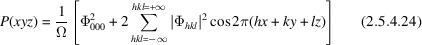and their analysis on the basis of heavy-atom methods, superposition methods and so on (see Chapter 2.3). Direct methods are also used (Dorset et al., 1979). Thus the phases of structure factors are calculated and assigned to the observed moduli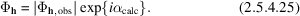The distribution of the potential in the unit cell, and, thereby, the arrangement in it of atoms (peaks of the potential) are revealed by the construction of three-dimensional Fourier series of the potential (see also Chapter 1.3)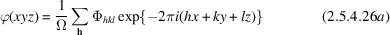or projections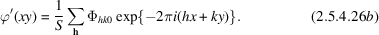The general formulae (2.5.4.26a)and (2.5.4.26b)transform, according to known rules, to the expressions for each space group (see IT I, 1952). If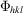are expressed in V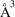and the volume Ω or the cell area S inand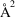, respectively, then the potential ϕ is obtained directly in volts, while the projection of the potentialis in V Å. The amplitudesare reduced to an absolute scale either according to a group of strong reflections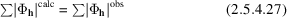or using the Parseval equality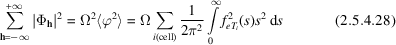or Wilson's statistical method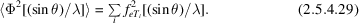The term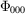defines the mean inner potential of a crystal, and is calculated from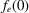[(2.5.4.13), (2.5.4.19)]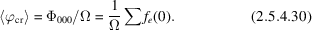The Fourier series of the potential in EDSA possess some peculiarities (Vainshtein, 1954, 1964) which make them different from the electron-density Fourier series in X-ray analysis. Owing to the peculiarities in the behaviour of the atomic amplitudes (2.5.4.13), which decrease more rapidly with increasing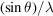compared with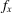, the peaks of the atomic potential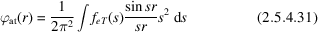are more blurred' and exhibit a larger half-width than the electron-density peaks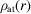. On average, this half-width corresponds to the resolution' of an electron-diffraction pattern – about 0.5 Å or better. The potential in the maximum (`peak height') does not depend as strongly on the atomic number as in X-ray analysis: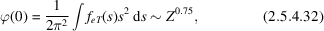while in X-ray diffraction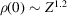. In such a way, in EDSA the light atoms are more easily revealed in the presence of heavy atoms than in X-ray diffraction, permitting, in particular, hydrogen atoms to be revealed directly without resorting to difference syntheses as in X-ray diffraction. Typical values of the atomic potential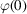(which depend on thermal motion) in organic crystals are: H ∼ 35, C ∼ 165, O 215 V; in Al crystals 330 V, in Cu crystals 750 V.

The EDSA method may be used for crystal structure determination, depending on the types of electron-diffraction patterns, for crystals containing up to several tens of atoms in the unit cell. The accuracy in determination of atomic coordinates in EDSA is about 0.01–0.005 Å on average. The precision of EDSA makes it possible to determine accurately the potential distribution, to investigate atomic ionization, to obtain values for the potential between the atoms and, thereby, to obtain data on the nature of the chemical bond.

If the positions in the cell are occupied only partly, then the measurement of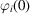gives information on population percentage.

There is a relationship between the nuclear distribution, electron density and the potential as given by the Poisson equation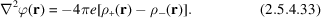This makes it possible to interrelate X-ray diffraction, EDSA and neutron-diffraction data. Thus for the atomic amplitudes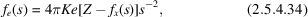where Z is the nuclear charge andthe X-ray atomic scattering amplitude, and for structure amplitudes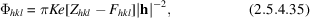where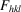is the X-ray structure amplitude of the electron density of a crystal and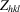is the amplitude of scattering from charges of nuclei in the cell taking into account their thermal motion. The valuescan be calculated easily from neutron-diffraction data, since the charges of the nuclei are known and the experiment gives the parameters of their thermal motion.

In connection with the development of high-resolution electron-microscopy methods (HREM) it has been found possible to combine the data from direct observations with EDSA methods. However, EDSA permits one to determine the atomic positions to a greater accuracy, since practically the whole of reciprocal space with 1.0–0.4 Å resolution is used and the three-dimensional arrangement of atoms is calculated. At the same time, in electron microscopy, owing to the peculiarities of electron optics and the necessity for an objective aperture, the image of the atoms in a crystal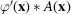is a convolution, with the aperture function blurring the image up to 1.5–2 Å resolution. In practice, in TEM one obtains only the images of the heaviest atoms of an object. However, the possibility of obtaining a direct image of a structure with all the defects in the atomic arrangement is the undoubted merit of TEM.

### ReferencesInternational Tables for X-ray Crystallography (1952). Vol. I. Symmetry groups. Birmingham: Kynoch Press. (Present distributor Kluwer Academic Publishers, Dordrecht.)Google ScholarDorset, D. L., Jap, B. K., Ho, M.-H. & Glaeser, R. M. (1979). Direct phasing of electron diffraction data from organic crystals: the effect of n-beam dynamical scattering. Acta Cryst. A35, 1001–1009.Google ScholarVainshtein, B. K. (1954). On the studies of crystal lattice potential by electron diffraction. Tr. Inst. Krist. Akad. Nauk SSSR, 9, 259–276. (In Russian.)Google ScholarVainshtein, B. K. (1964). Structure analysis by electron diffraction. Oxford: Pergamon Press.Google Scholar